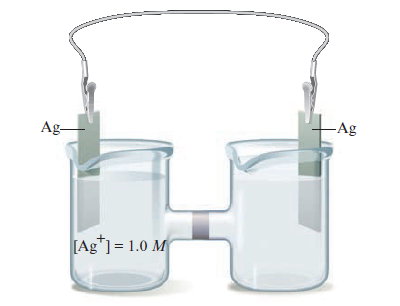# Problem: Consider a concentration cell similar to the one shown below, except that both electrodes are made of Ni and in the left-hand compartment [Ni2+] = 1.0 M. Calculate the cell potential at 25°C when the concentration of Ni2+ in the compartment on the right has each of the following values.c. 0.10 MFor each case, also identify the cathode, anode, and the direction in which electrons flow.

91% (21 ratings)
###### Problem Details

Consider a concentration cell similar to the one shown below, except that both electrodes are made of Ni and in the left-hand compartment [Ni2+] = 1.0 M. Calculate the cell potential at 25°C when the concentration of Ni2+ in the compartment on the right has each of the following values.

c. 0.10 MFor each case, also identify the cathode, anode, and the direction in which electrons flow.

Frequently Asked Questions

What scientific concept do you need to know in order to solve this problem?

Our tutors have indicated that to solve this problem you will need to apply the The Nernst Equation concept. You can view video lessons to learn The Nernst Equation. Or if you need more The Nernst Equation practice, you can also practice The Nernst Equation practice problems.

What textbook is this problem found in?

Our data indicates that this problem or a close variation was asked in Chemistry: An Atoms First Approach - Zumdahl Atoms 1st 2nd Edition. You can also practice Chemistry: An Atoms First Approach - Zumdahl Atoms 1st 2nd Edition practice problems.